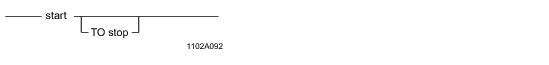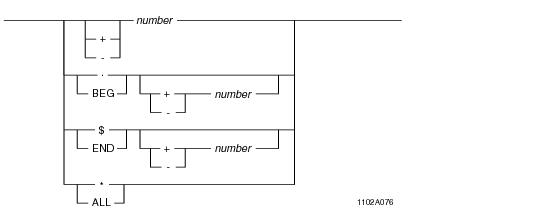# 15.00 - Specifying Display Ranges (ordinalrange) - Teradata Database

prodname
vrm_release
15.00
category
Configuration
featnum
B035-1180-015K

### Specifying Display Ranges (ordinalrange)

The ordinalrange parameter is used to specify a range of objects relative to the current position to be displayed.

ordinalrange can be used on the following commands:

• DISPLAY
• TABLE
• WAL
•
 When used on the … ordinalrange … DISPLAY command restricts or expands the initial context, producing a different resulting context. DISPLAY shows the objects in the new context. Filer saves the context from a previous command, and the ordinalrange is interpreted with respect to this initial context. The initial context for the DISPLAY command can be a physical row, subtable, CI, CID, DB, DBD, MI, WAL log record, WCI, WCID, WDB, WDBD, WMI, or a range thereof (except for the MI and WMI which are singular contexts). TABLE command restricts the range of physical rows to display, but does not influence the resulting context. An implicit initial context consisting of the physical rows of the table, and the ordinalrange is interpreted with respect to this context. The initial context for the TABLE command is always this same row range context. No matter what is displayed, the resulting context is always a subtable rather than a row range. WAL command restricts the WAL log records that are displayed. The initial context is from the first WAL log record to the last, and the ordinalrange is interpreted with respect to this context. The resulting context is a WAL log record or WAL log record range; therefore, the resulting context matches the records selected.

Note: The Filer online help refers to ordinalrange as rangeopt, but the usage is the same.

ordinalrange input is as follows:start and stop are defined as follows:Syntax Element Description BEG BEG always refers to the first object in the context. If the context is singular, BEG and END refer to the same object. END END always refers to the last object in the context. If the context is singular, BEG and END refer to the same object. +/- number The meaning of +/- number without BEG/END depends on whether it is used on a DISPLAY, TABLE, or WAL command: DISPLAY command + number is equivalent to END + number, and – number is equivalent to BEG - number, as described below: A + number is a displacement from the last object in the context, expanding the context beyond the last object by the specified number of objects. A - number is a displacement from the first object in the context, expanding the context before the first object by the specified number of objects. TABLE command + number is equivalent to BEG + number - 1, and – number is equivalent to END-number, as described below: A + number is an ordinal row number, where 1 is the first physical row. A - number is a backwards displacement from the last physical row, so that -1 is the second to the last physical row. The exception is if the TABLE command uses a wildcard for the type part of the tid. In this case, the resultant context is special (nothing), and the display is a multiple subtable display, where each subtable is constrained by the ordinal range. For each subtable, the BEG/END +/- number rules apply, except that the – number construct is not allowed. WAL command + number is equivalent to BEG + number - 1, and – number is equivalent to END-number, as described below: A + number is an ordinal record number, where 1 is the first WAL log record. A - number is a backwards displacement from the last record, so that -1 is the second to the last WAL log record. For examples, see “DISPLAY” on page 127, “TABLE” on page 214, and “WAL” on page 223. BEG/END +/- number Allows specifying an object relative to the first or last object of a context. When used on a DISPLAY command, BEG/END +/- number can be used to expand or contract a context. When used on a TABLE or WAL command, BEG/END +/- number can only restrict the initial context for display purposes. . If the current position encompasses a range of objects, then start or the period (.) means from the first object in the range. \$ If the current position encompasses a range of objects, then stop or the dollar sign (\$) means from the last object in the range. */ALL If you specify an asterisk (*) or ALL as the end field, ALL is translated into an end field. After an operation involving ordinalrange, the context, including the currently selected object, is adjusted to be these new objects just acted upon by this command.